In :
%matplotlib inline

import pandas as pd
import numpy as np
import matplotlib.pyplot as plt
import seaborn as sns

In :
# Get cleaned data

Out:
Unnamed: 0 timestamp likeable surprising q1 q2 q3 q4 q5 q6 q7 q8 q9 q10 age gender edu group
0 0 2019/01/10 11:13:00 AM EST 7.0 3.0 -88.0 15.0 1.0 15.0 33.0 -80.0 -33.0 58.0 -50.0 33.0 27.0 m 4yr 0
1 1 2019/01/10 11:32:59 AM EST 4.0 5.0 -30.0 30.0 1.0 14.0 10.0 -50.0 -5.0 51.0 -30.0 10.0 18.0 m high 0
2 2 2019/01/10 11:33:55 AM EST 3.0 3.0 -85.0 20.0 3.0 35.0 36.0 -86.0 100.0 50.0 -30.0 300.0 21.0 m high 0
3 3 2019/01/10 11:34:50 AM EST 7.0 3.0 -88.0 30.0 1.0 15.0 30.0 -88.0 -33.0 56.0 -51.0 300.0 45.0 m 4yr 0
4 4 2019/01/10 11:35:08 AM EST 7.0 7.0 -86.0 32.0 1.0 26.0 32.0 -36.0 16.0 56.0 -17.0 40.0 17.0 m none 0
In :
# Time to calculate THE SCORES!

# See my pre-registration for the rationale behind the formula:
# https://blog.ncase.me/my-experiment-pre-registration/

# For each Q:
# y = _/\_-shaped, peaks at 1.0 +/- 0.5
#   = max( 0, 1-2*abs(x-1) )

# 1 (poverty): -88
# 2 (air pollution): +25
# 3 (violence): +1
# 4 (mental): +15
# 5 (heart): +32
# 6 (nukes): -84
# 7 (suicide): -33
# 8 (democracy): +56
# 9 (fertility): -51
# 10 (CO2): +302

'q1': -88,
'q2': +25,
'q3': +1,
'q4': +15,
'q5': +32,
'q6': -84,
'q7': -33,
'q8': +56,
'q9': -51,
'q10': +302
}

def calculate_score(row):
total_points = 0

# for each q...
for i in range(10):
q_name = "q" + str(i+1)
guess = row[q_name]
y = max( 0, 1-2*abs(x-1) )
total_points += y

# gimme!

data['score'] = data.apply(lambda row: calculate_score(row), axis=1)

Out:
Unnamed: 0 timestamp likeable surprising q1 q2 q3 q4 q5 q6 q7 q8 q9 q10 age gender edu group score
0 0 2019/01/10 11:13:00 AM EST 7.0 3.0 -88.0 15.0 1.0 15.0 33.0 -80.0 -33.0 58.0 -50.0 33.0 27.0 m 4yr 0 7.931618
1 1 2019/01/10 11:32:59 AM EST 4.0 5.0 -30.0 30.0 1.0 14.0 10.0 -50.0 -5.0 51.0 -30.0 10.0 18.0 m high 0 3.655042
2 2 2019/01/10 11:33:55 AM EST 3.0 3.0 -85.0 20.0 3.0 35.0 36.0 -86.0 100.0 50.0 -30.0 300.0 21.0 m high 0 5.183139
3 3 2019/01/10 11:34:50 AM EST 7.0 3.0 -88.0 30.0 1.0 15.0 30.0 -88.0 -33.0 56.0 -51.0 300.0 45.0 m 4yr 0 9.366517
4 4 2019/01/10 11:35:08 AM EST 7.0 7.0 -86.0 32.0 1.0 26.0 32.0 -36.0 16.0 56.0 -17.0 40.0 17.0 m none 0 4.394545
In :
import math
from scipy.stats import ttest_ind

# Calculate effect size, p-value
# for Score, Likeable, and Surprising
control = data[data["group"]==0]
experimental = data[data["group"]==1]
metrics = {
"score": {},
"likeable": {},
"surprising": {}
}

# Standardized Effect Size (Cohen's d)
def cohend_col(col):
d1 = experimental[col]
d2 = control[col]
return cohend(d1,d2)
def cohend(d1, d2):
n1, n2 = len(d1), len(d2)
s1, s2 = np.var(d1, ddof=1), np.var(d2, ddof=1)
s = math.sqrt(((n1 - 1) * s1 + (n2 - 1) * s2) / (n1 + n2 - 2))
u1, u2 = np.mean(d1), np.mean(d2)
return (u1 - u2) / s
metrics["score"]["d"] = cohend_col("score")
metrics["likeable"]["d"] = cohend_col("likeable")
metrics["surprising"]["d"] = cohend_col("surprising")

# P-Value
def get_p(col):
return ttest_ind(experimental[col], control[col]).pvalue
metrics["score"]["p"] = get_p("score")
metrics["likeable"]["p"] = get_p("likeable")
metrics["surprising"]["p"] = get_p("surprising")

# Let's see it
metrics

Out:
{'score': {'d': -0.004416331890078157, 'p': 0.9291907434347007},
'likeable': {'d': 0.5496709310749239, 'p': 1.8023150053255885e-27},
'surprising': {'d': 0.5126581126827132, 'p': 3.2600809813287064e-24}}
In :
import matplotlib.pyplot as plt
import seaborn as sns
sns.set_style("dark")

# Draw histograms for Score, Likeable, Surprising
for key, stats in metrics.items():

sns.distplot(control[key], label="control")
sns.distplot(experimental[key], label="experimental")

d_string = "{0:.3f}".format(stats["d"])
p_string = "{0:.3f}".format(stats["p"])

plt.title(key.title()+" (d="+d_string+", p="+p_string+")")
plt.legend()
plt.show()

/Users/ncase/anaconda3/lib/python3.7/site-packages/scipy/stats/stats.py:1713: FutureWarning: Using a non-tuple sequence for multidimensional indexing is deprecated; use arr[tuple(seq)] instead of arr[seq]. In the future this will be interpreted as an array index, arr[np.array(seq)], which will result either in an error or a different result.
return np.add.reduce(sorted[indexer] * weights, axis=axis) / sumval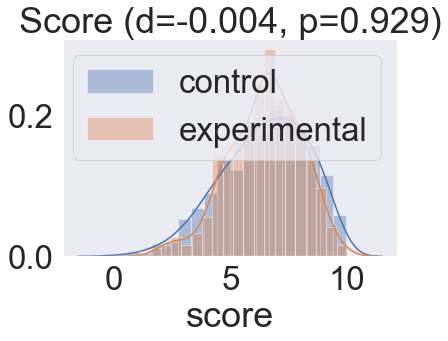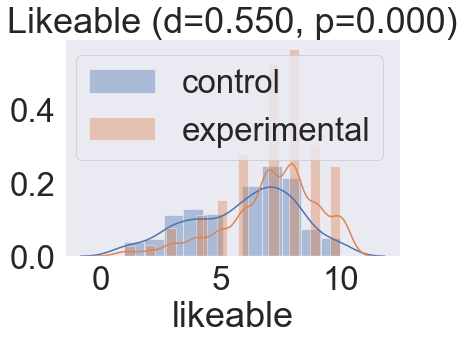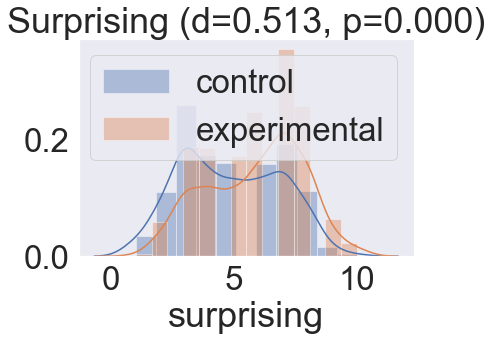In :
# Use resampling to get mean differences between experimental & control
diffs = {
"score": {"resamples":[]},
"likeable": {"resamples":[]},
"surprising": {"resamples":[]}
}
effects = {
"score": {"resamples":[]},
"likeable": {"resamples":[]},
"surprising": {"resamples":[]}
}
resample_n = 1000
for i in range(resample_n):

# Resample
resampled_data = data.sample(n=data.shape, replace=True, random_state=i)
resampled_control = resampled_data[resampled_data["group"]==0]
resampled_experimental = resampled_data[resampled_data["group"]==1]

# Simulate calculating the absolute mean diff + effect size
for key in diffs:
diffs[key]["resamples"].append( resampled_experimental[key].mean() - resampled_control[key].mean() )
effects[key]["resamples"].append( cohend(resampled_experimental[key], resampled_control[key]) )

# Get means & 95% bounds on resamples
for key in diffs:
for d in [diffs, effects]:
resamples = d[key]["resamples"]
mean = np.mean(resamples)
d[key]["mean"] = mean
d[key]["lower_bound"] = mean - np.percentile(resamples, 2.5)
d[key]["upper_bound"] = np.percentile(resamples, 100-2.5) - mean

# Generate plots
sns.set_style("darkgrid")
def generate_diffs(d, col):
return [d["score"][col], d["likeable"][col], d["surprising"][col]]
def generate_plot(d, title):

# Generate data
x = generate_diffs(d,"mean")
y = range(1,4)
lower_error = generate_diffs(d,"lower_bound")
upper_error = generate_diffs(d,"upper_bound")
error = [lower_error, upper_error]

# Create & show the plot
sns.set(font_scale=3)
fig, ax = plt.subplots(figsize=(30,4))
ax.errorbar(x, y, xerr=error, fmt='o', markersize=30, elinewidth=6)
ax.set_title(title)
ax.set_ylim(0.5,3.5) # make it pretty
plt.yticks(y, ["Memory", "Likeable", "Surprising"])
plt.axvline(0, linewidth=6, color=(0,0,0,0.3))
plt.show()

# Plot ABSOLUTE DIFFS:
generate_plot(diffs, "Absolute difference between Experimental & Control (95% CI from bootstrapping)")

# Plot STANDARDIZED EFFECT SIZES:
generate_plot(effects, "Standardized effect sizes (Cohen's d) of Experimental vs Control (95% CI from bootstrapping)")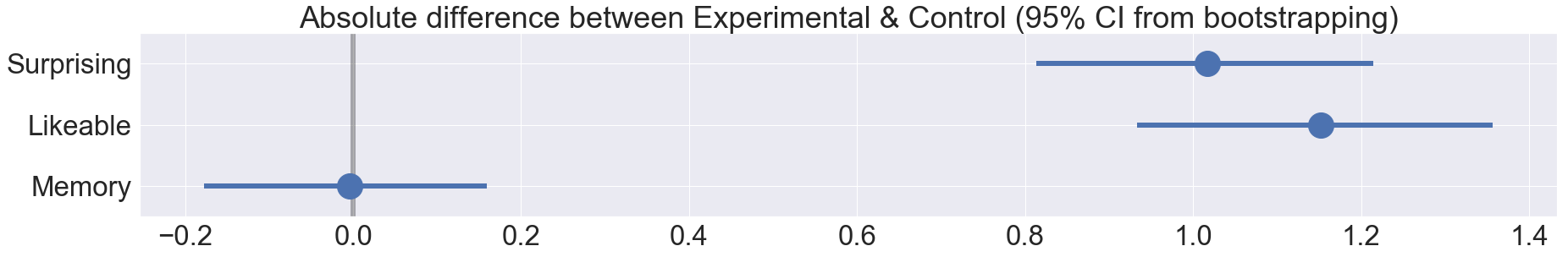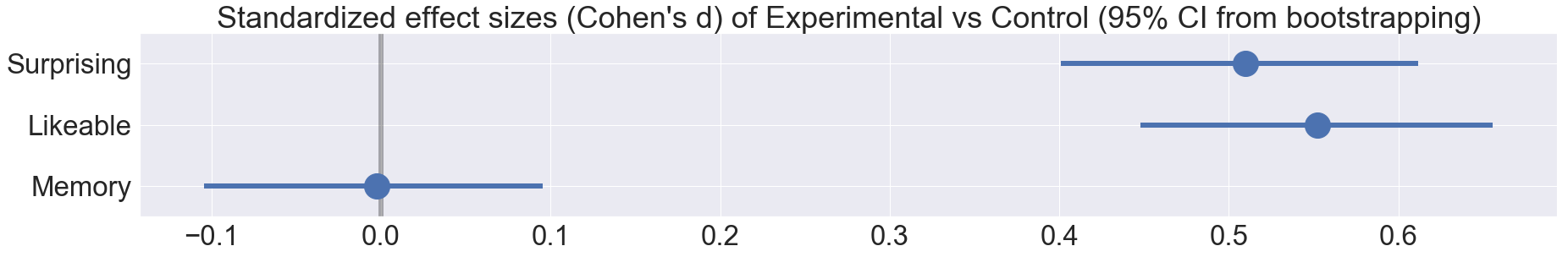In [ ]: